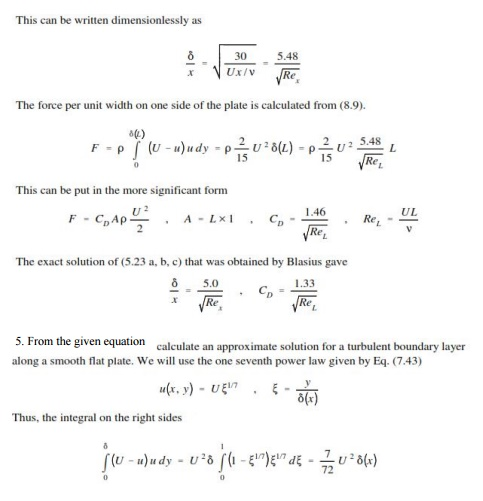Home | | Mechanics of Fluids | Mechanics Of Fluids- Formation of the boundary layer

# Mechanics Of Fluids- Formation of the boundary layer

The fluid near the top of the boundary layer is dragging the fluid nearer to the solid surface along. The mechanism for this dragging may be one of two types.

Formation of the boundary layer

Previously we noted that the boundary layer grows from zero when a fluid starts to flow over a solid surface. As is passes over a greater length more fluid is slowed by friction between the fluid layers close to the boundary. Hence the thickness of the slower layer increases.

The fluid near the top of the boundary layer is dragging the fluid nearer to the solid surface along. The mechanism for this dragging may be one of two types:

The first type occurs when the normal viscous forces (the forces which hold the fluid together) are large enough to exert drag effects on the slower moving fluid close to the solid boundary. If the boundary layer is thin then the velocity gradient normal to the surface, (du/dy), is large so by Newton's law of viscosity the shear stress, = m (du/dy), is also large. The corresponding force may then be large enough to exert drag on the fluid close to the surface.

As the boundary layer thickness becomes greater, so the velocity gradient become smaller and the shear stress decreases until it is no longer enough to drag the slow fluid near the surface along. If this viscous force was the only action then the fluid would come to a rest.

It, of course, does not come to rest but the second mechanism comes into play. Up to this point the flow has been laminar and Newton's law of viscosity has applied. This part of the boundary layer is known as the laminar boundary layer

The viscous shear stresses have held the fluid particles in a constant motion within layers. They become small as the boundary layer increases in thickness and the velocity gradient gets smaller. Eventually they are no longer able to hold the flow in layers and the fluid starts to rotate.This causes the fluid motion to rapidly becomes turbulent. Fluid from the fast moving region moves to the slower zone transferring momentum and thus maintaining the fluid by the wall in motion. Conversely, slow moving fluid moves to the faster moving region slowing it down. The net effect is an increase in momentum in the boundary layer. We call the part of the boundary layer the turbulent boundary layer.

At points very close to the boundary the velocity gradients become very large and the velocity gradients become very large with the viscous shear forces again becoming large enough to maintain the fluid in laminar motion. This region is known as the laminar sub-layer. This layer occurs within the turbulent zone and is next to the wall and very thin - a few hundredths of a mm.

Surface roughness effect

Despite its thinness, the laminar sub-layer can play a vital role in the friction characteristics of the surface.

This is particularly relevant when defining pipe friction - as will be seen in more detail in the level 2 module. In turbulent flow if the height of the roughness of a pipe is greater than the thickness of the laminar sub-layer then this increases the amount of turbulence and energy losses in the flow. If the height of roughness is less than the thickness of the laminar sub-layer the pipe is said to be smooth and it has little effect on the boundary layer.

In laminar flow the height of roughness has very little effect

Boundary layers in pipes

As flow enters a pipe the boundary layer will initially be of the laminar form. This will change depending on the ration of inertial and viscous forces; i.e. whether we have laminar (viscous forces high) or turbulent flow (inertial forces high).

From earlier we saw how we could calculate whether a particular flow in a pipe is laminar or turbulent using the Reynolds number.

Re = rud / m

(r = density   u = velocity   m = viscosity  d = pipe diameter)

Laminar flow:                          Re < 2000

Transitional flow: 2000 < Re < 4000

Turbulent flow:                        Re > 4000If we only have laminar flow the profile is parabolic - as proved in earlier lectures - as only the first part of the boundary layer growth diagram is used. So we get the top diagram in the above figure.

If turbulent (or transitional), both the laminar and the turbulent (transitional) zones of the boundary layer growth diagram are used. The growth of the velocity profile is thus like the bottom diagram in the above figure.

Once the boundary layer has reached the centre of the pipe the flow is said to be fully developed. (Note that at this point the whole of the fluid is now affected by the boundary friction.)

The length of pipe before fully developed flow is achieved is different for the two types of flow. The length is known as the entry length.

Laminar flow entry length   120  diameter

Turbulent flow entry length   60  diameterStudy Material, Lecturing Notes, Assignment, Reference, Wiki description explanation, brief detail
Civil : Mechanics Of Fluids : Boundary Layer : Mechanics Of Fluids- Formation of the boundary layer |